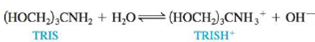# Tris(hydroxymethyl)aminomethane, commonly called TRIS or Trizma, is often used as a buffer in biochemical studies. Its buffering range is pH 7 to 9, and K b is 1.19 × 10 −6 for the aqueous reaction a. What is the optimal pH for TRIS buffers? b. Calculate the ratio [TRIS]/[TRISH + ] at pH = 7.00 and at pH = 9.00. c. A buffer is prepared by diluting 50.0 g TRIS base and 65.0 g TRIS hydrochloride (written as TRISHCl) to a total volume of 2.0 L. What is the pH of this buffer? What is the pH after 0.50 mL of 12 M HCl is added to a 200.0-mL portion of the buffer?### Chemistry: An Atoms First Approach

2nd Edition
Steven S. Zumdahl + 1 other
Publisher: Cengage Learning
ISBN: 9781305079243

#### Solutions

Chapter
Section### Chemistry: An Atoms First Approach

2nd Edition
Steven S. Zumdahl + 1 other
Publisher: Cengage Learning
ISBN: 9781305079243
Chapter 14, Problem 83AE
Textbook Problem
11 views

## Tris(hydroxymethyl)aminomethane, commonly called TRIS or Trizma, is often used as a buffer in biochemical studies. Its buffering range is pH 7 to 9, and Kb is 1.19 × 10−6 for the aqueous reactiona. What is the optimal pH for TRIS buffers?b. Calculate the ratio [TRIS]/[TRISH+] at pH = 7.00 and at pH = 9.00.c. A buffer is prepared by diluting 50.0 g TRIS base and 65.0 g TRIS hydrochloride (written as TRISHCl) to a total volume of 2.0 L. What is the pH of this buffer?What is the pH after 0.50 mL of 12 M HCl is added to a 200.0-mL portion of the buffer?

(a)

Interpretation Introduction

Interpretation:

Tris(hydroxymethyl)aminomethane, commonly known as TRIS is given to be a commonly used buffer in the biochemical studies. Its buffering range (79) and its Kb is given. Various questions related to this are to be answered.

Concept introduction:

The relation between pH and pKa is given by Henderson-Hasselbach equation. According to this equation,

pH=pKa+log[Salt][Acid]

### Explanation of Solution

Explanation

Given

The Kb for TRIS is 1.19×106 .

The value of Ka is calculated by the formula,

Ka=KwKb

Where,

• Kw is the ionic product of water (1.0×1014) .
• Ka is the acid dissociation constant.
• Kb is the base ionization constant.

Substitute the value of Kw and Kb in the above expression.

Ka=1.0×10141.19×106=8.40×10-9_

Therefore, the Ka of TRIS is 8

(b)

Interpretation Introduction

Interpretation:

Tris(hydroxymethyl)aminomethane, commonly known as TRIS is given to be a commonly used buffer in the biochemical studies. Its buffering range (79) and its Kb is given. Various questions related to this are to be answered.

Concept introduction:

The relation between pH and pKa is given by Henderson-Hasselbach equation. According to this equation,

pH=pKa+log[Salt][Acid]

(c)

Interpretation Introduction

Interpretation:

Tris(hydroxymethyl)aminomethane, commonly known as TRIS is given to be a commonly used buffer in the biochemical studies. Its buffering range (79) and its Kb is given. Various questions related to this are to be answered.

Concept introduction:

The relation between pH and pKa is given by Henderson-Hasselbach equation. According to this equation,

pH=pKa+log[Salt][Acid]

### Still sussing out bartleby?

Check out a sample textbook solution.

See a sample solution

#### The Solution to Your Study Problems

Bartleby provides explanations to thousands of textbook problems written by our experts, many with advanced degrees!

Get Started

Find more solutions based on key concepts
Eating disorders are common only in cultures where extreme thinness is an ideal. Who in society do you think se...

Nutrition: Concepts and Controversies - Standalone book (MindTap Course List)

9-82 What is the ground state of a nucleus?

Introduction to General, Organic and Biochemistry

Review. A Chinook salmon can swim underwater at 3.58 m/s, and it can also jump vertically upward, leaving the w...

Physics for Scientists and Engineers, Technology Update (No access codes included)

What is meant by westward intensification? Why are western boundary currents so fast and deep?

Oceanography: An Invitation To Marine Science, Loose-leaf Versin